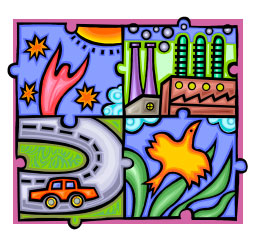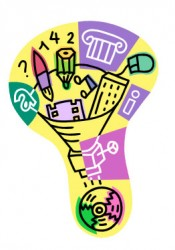Getting Started on Your Science Fair Project

The Scientific Method

A science project uses experimentation and the scientific method to determine the answer or best solution to a scientific problem. It is important to understand the scientific method before starting a project. The scientific method is the standard tool that scientists use to find the answers to questions.

The scientific method involves the following steps:
• Define the question
• Gather information and resources
• Form a hypothesis
• Perform experiment
• Collect, analyze and interpret data (to draw conclusions that can serve as a starting point for new hypothesis)
• Project conclusion (Publish results)Define the QuestionThe very first thing to do in a science project is define the question. What is it that you want to answer? Example: Will soaking chile pepper seeds in water help them germinate faster? You can actually turn this one idea into several different ideas. Will soaking chile pepper seeds in saltwater help them germinate faster? Will soaking chile pepper seeds in vinegar help them germinate faster?

Gather Information and Resources

Project research is the process of collecting information from knowledgeable sources such as books, magazines, software, librarians, teachers, parents, scientists, or other professionals. Your first research is used to select a project topic. The information you gather is to help you understand the topic, express a problem, propose a hypothesis, and design an experiment. It is also data collected from exploratory experimentation.Form a Hypothesis

A hypothesis is an idea about the solution to a problem, based on knowledge and research from previous experiments or problems. While the hypothesis is a single statement, it is the key to a successful project. All your project research is done with the goal of expressing a problem, proposing an answer to it (the hypothesis), and designing project experimentation. All of your project experimenting will be performed to test the hypothesis. The hypothesis should make a claim about how two factors relate. For example, in the following sample hypothesis, the two relating factors are soaking solution and germination rates. Here is one example of a hypothesis: “By using a soaking solution, we will be able to determine how quickly a chile pepper seed will germinate.”

Perform ExperimentProject experimentation is the process of testing a hypothesis. The things that have an effect on the experiment are called variables. There are three kinds of variables that you need to identify in your experiments; independent, dependent, and controlled. The independent variable is the variable you purposely manipulate (change). The dependent variable is the variable that is being observed, which changes in response to the independent variable. The variables that are not changed are called controlled variables. A control is a test in which the independent variable is kept constant in order to measure changes in the dependent variable. In a control, all variables are identical to the experimental setup your original setup except for the independent variable. Factors that are identical in both the experimental setup and the control setup are the controlled variables. Here is where you will start by asking a question, gathering materials that will help you answer the question and coming up with a procedure for your experiment.

Collect, Analyze and Interpret Data

Before you can state the results of an experiment, you must first organize all the data collected during experimentation. Numbers, called “raw data,” have little meaning unless you organize and label them. Data from each experiment needs to be written down in an orderly way.

Project Conclusion

The project conclusion is a summary of the results of the project experimentation and a statement of how the results relate to the hypothesis. You should include reasons for experimental results that are contrary to the hypothesis. If applicable, the conclusion can end by giving ideas for further testing.A Chile Pepper Institute publication, New Mexico State University © 2007